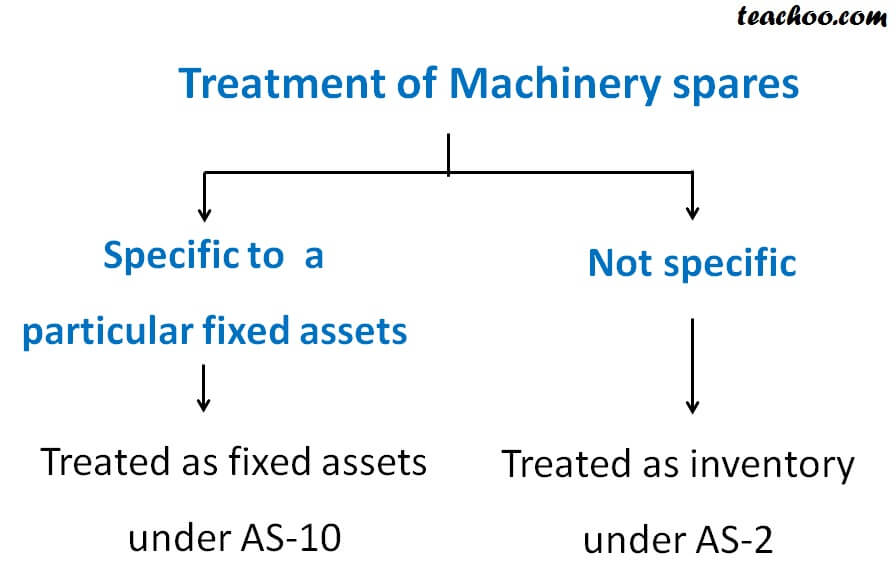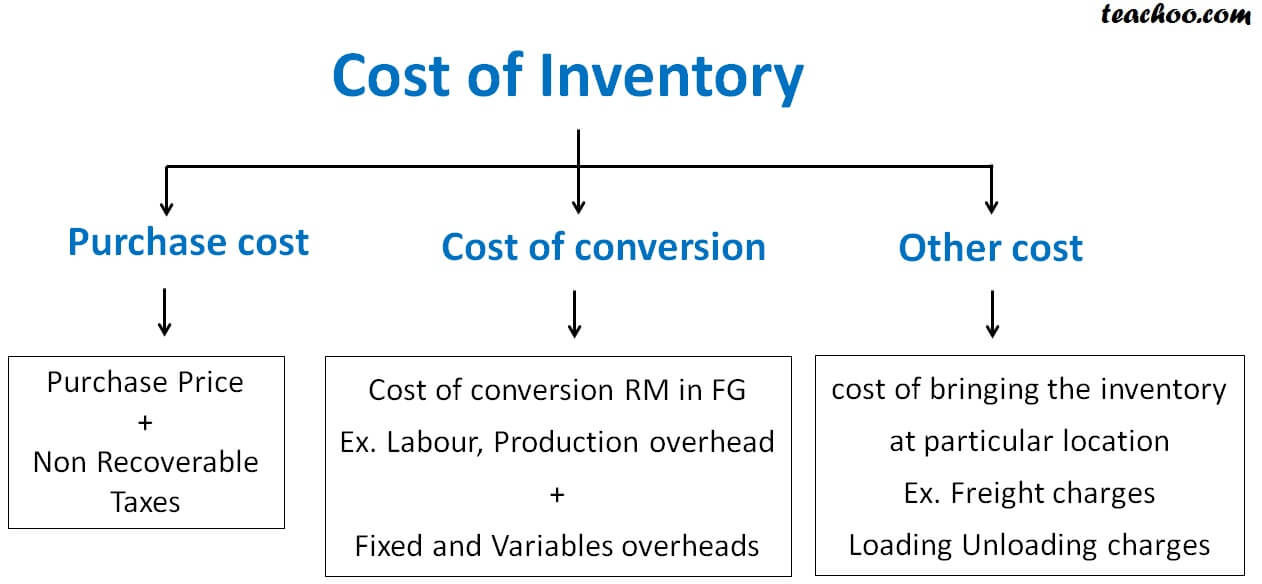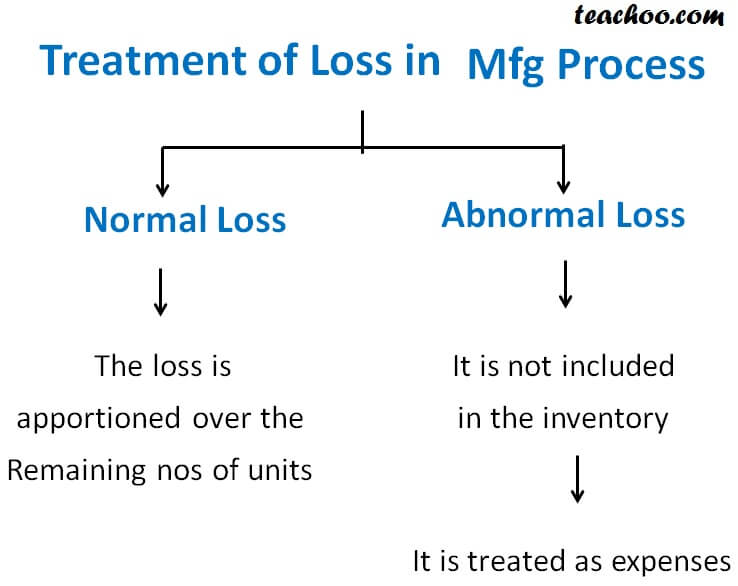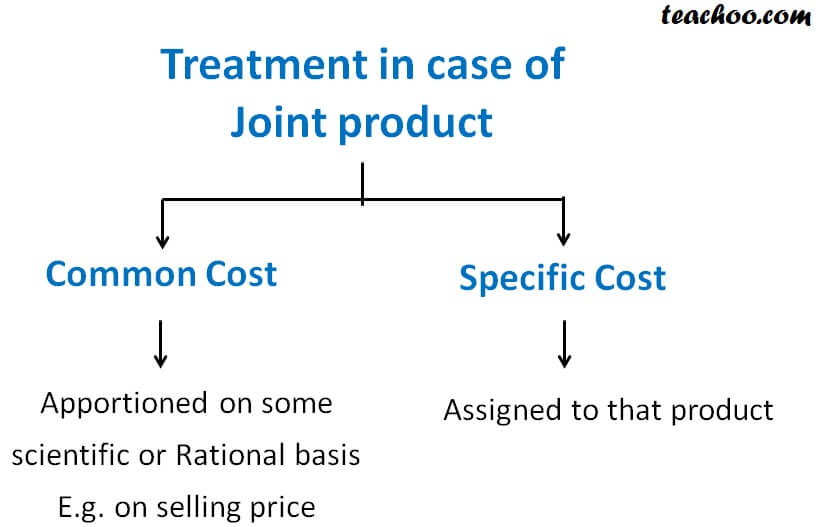Accounting Standards

Accounts and Finance
Accounts Theory

Types of Inventories :

1. Finished Goods
2. Raw Material
3. Work in Progress
4. Consumable stores and sparesItems not included in the cost of Inventory

1. Borrowing cost
2. Selling & distribution cost
4. Abnormal lossIllustration : Co.  purchased 1000 pcs at Rs.100 each . out of this 5% is normal loss. No item was sold. A t the end of year the co. only had 920 pcs in hand . inventory value ?

Normal Loss    =  1000 X 5%   =  50

Cost per Piece  =  1000 X 100 / 950   =  105.26

Abnormal loss = 950 – 920 = 30

Cost of abnormal loss = 30 X 105.26 =  3157.80

Closing stock value = 920 X 105.26  = 96839.20

Joint Product : No major difference in value between main product or other product

By product : Major difference in value between main product and by product such as finished good and scrap

#### Treatment in case of Joint product

While making joint product there are certain joint cost like cost of material , conversion etc. Also there are some specific cost to a joint product like packing cost#### Treatment of By - Product

The net realisable value of by product is reduced from the value of main product. Such as NRV of scrap is lessed from value of finished goods.

Valuation of Inventory as per AS-2 on the Balance sheet date?

They are valued at

1. Cost  (Whichever is less)
2. Net Realisable value (Whichever is less)

#### Steps

Step 1 : Calculate cost (purchase price + conversion cost + other cost – abnormal loss)

Step 2 :  Calculate Net realisable value (Selling price – further cost of selling)

Example 1 : the co. had inventory whose cost of Rs.80000. It can sold for Rs.90000. cost of inventory ?

In above case , cost is less than NRV so cost Rs.80000 will be cost of inventory

Example 2 : suppose  it can be sold Rs.90000 but co. has to pay  20% commission

Net realisable value = selling price – commission

90000 – 18000 = 72000

Cost = 80000

In above case, NRV is less than cost so NRV will be Inventory value that is 72000

Example 3 : co. had raw material cost Rs.100000 if they are to be manufactured  the cost of manufacture would be 40000 and then it could be sold for Rs.130000

Cost  = 100000

NRV = Selling price – further cost

=   130000 – 40000  = 90000

NRV is less than the cost so NRV 90000 would be value of inventory

Example 4 : suppose in example 3 the raw material can be sold as it is 93000

Raw material cost  = 100000       NRV = 93000

Inventory value = 93000

#### Guidance note to CENVAT

1. Value of input cenvat not included in inventory
2. In case of finished goods Excise duty liability arises on manufacturer but is payable on the sale of good so in this case  increase the value of inventory by excise and correspondingly make a provision for excise duty

Method of valuation of Inventory AS-2

Normally FIFO (first in first out) and weighted average is used not LIFO (last in first out)

Items not covered under As-2

1. Agriculuture
2. construction (AS-7)
3. Service contract
4. Mines
5. financial Instrument like shares, debentures

Learn in your speed, with individual attention - Teachoo Maths 1-on-1 Class